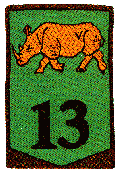# 13

This number is a prime.

Just showing those entries submitted by 'Rivera': (Click here to show all)Photo credit: Patrick KikThe square of the prime 13 is 169 and 169 is the concatenation of the squares of two consecutive numbers 4 and 3. This is the largest prime known with this property. [Rivera]13 is the smallest prime that remains prime when its digits d are changed to d^n, for n = 2, 3 and 4, one at a time: for n=2, 13->19; for n=3, 13->127; for n=4, 13->181. [Rivera]